## Thursday, March 14, 2013

### Clustering in Oracle Data Miner-Part 5

This is a the fifth and final blog post on building and using Clustering in Oracle Data Miner. The following outlines the contents of each post in this series on Clustering.

1. The first part we looked at what clustering features exist in ODM and how to setup the data that we will be using in the examples
2. The second part will focus on how to building Clusters in ODM .
3. The third post will focus on examining the clusters produced by ODM and how to use the Clusters to apply to new data using ODM.
4. The fourth post will look at how you can build and evaluate a Clustering model using the ODM SQL and PL/SQL functions.
5. The fifth and final post will look at how you can apply your Clustering model to new data using the ODM SQL and PL/SQL functions.

Step 1 – What Clustering models do we have

In my previous post I gave the query to retrieve the clustering models that we have in our schema. Here it is again.

column model_name format a20
column mining_function format a20
column algorithm format a20
SELECT model_name,
mining_function,
algorithm,
build_duration,
model_size
FROM ALL_MINING_MODELS
WHERE mining_function = 'CLUSTERING';

This time we see that we have 3 cluster models. Our new model is called CLUSTER_KMEANS_MODEL.

column child format a40
column cluster_id format a25

select cluster_id,
record_count,
parent,
tree_level,
child
from table(dbms_data_mining.get_model_details_km('CLUS_KM_1_25'))

The following image shows all the clusters produced and we can see that we have the renamed cluster labels we set when we used the ODM tool.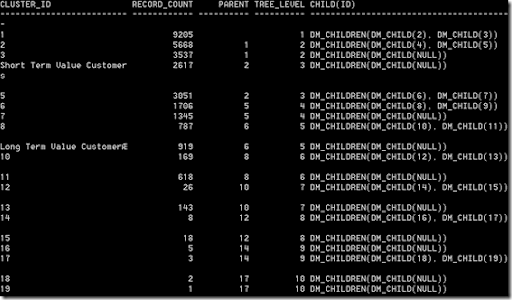Step 2 – Setting up the new data

There are some simple rules to consider when preparing the data for the cluster model. These really apply to all of the data mining algorithms.

- You will need to have the data prepared and in the same format as you used for building the model

- This will include the same table structure. Generally this should not be a problem. If you need to merge a number of tables to form a table with the correct format, the simplest method is to create a view.

- All the data processing for the records and each attribute needs to be completed before you run the apply function.

- Depending on the complexity of this you can either build this into the view (mentioned above), run some pl/sql procedures and create a new table with the output, etc.  I would strongly suggest that the minimum pre-processing you have to do on the data the simpler the overall process and implementation will be.

- The table or view must have one attribute for the CASE_ID. The CASE_ID is an attribute that is unique for each record. If the primary key of the table is just one attribute you can use this. If not then you will need to generate a new attribute that is unique. One way to do this is to concatenate each of the attributes that form the primary key.

Step 3 – Applying the Cluster model to new data – In Batch mode

There are two ways of using an Oracle Data Mining model in the database. In this section we will look at how you can run the cluster model to score data in a batch mode. What I mean by batch mode is that you have a table of data available and you want to score the data with what the model thinks their cluster will be.

To do this we need to run the APPLY function that is part of the DBMS_DATA_MINING package.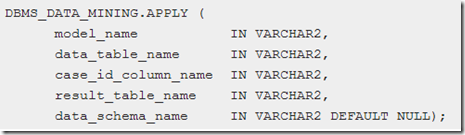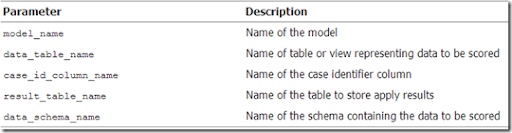For clustering we do not have CASE_ID, so we can leave this parameter NULL.

One of the parameters is called RESULT_TABLE_NAME. Using the DBMS_DATA_MINING.APPLY package and function, it looks to create a new table that will contain the outputs of the cluster scoring. This table (for the KMeans and O-Cluster algorithms) will contain three attributes.

CASE_ID       VARCHAR2/NUMBER
CLUSTER_ID    NUMBER
PROBABILITY   NUMBER

The table will have the CASE_ID. This is the effectively the primary key of the table.

If we take our INSURANCE_CUST_LTV table as the table containing the new data we want to score (Yes this is the same table we used to build the cluster model) and the CLUSTER_KMEANS_MODEL as the cluster model we want to use. The following codes show the APPLY function necessary to score the data.

BEGIN

DBMS_DATA_MINING.APPLY(
model_name          => 'CLUSTER_KMEANS_MODEL',
data_table_name     => 'INSURANCE_CUST_LTV',
case_id_column_name => 'CUSTOMER_ID',
result_table_name   => 'CLUSTER_APPLY_RESULT');
END;

On my laptop this took 3 second to complete. This involved scoring 15,342 records, creating the table CLUSTER_APPLY_RESULT and inserting 153,420 scored records into the table CLUSTER_APPLY_RESULT.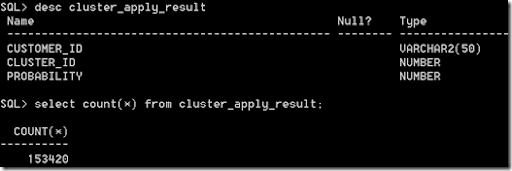Why did we get 10 times more records in our results table than we did in our source table ?

Using the batch mode i.e. using the DBMS_DATA_MINING.APPLY function it will create a record for each of the possible clusters that the record will belong too along with the probability of it belonging to that cluster. In our case we have built our clustering models based on 10 clusters.

In the following diagram we have a listing for two of the customers in our dataset, the clusters that have been assigned to them and the probability of that record/customer belonging to that cluster. We can then use this information to make various segmentation decisions based on the probabilities that each has for the various clusters.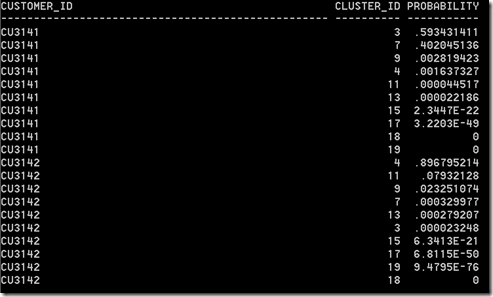Step 4 – Applying the Cluster model to new data – In Real-time mode

When we looked at applying a classification algorithm to new data we were able to use the PREDICTION SQL function. As clustering is an unsupervised data mining technique we will not be able to use the PREDICTION function.

Instead we have the functions CLUSTER_ID and CLUSTER_PROBABILITY.

CLUSTER_ID will tell us what cluster the record is most likely to belong too i.e. the cluster with the highest probability.

This is different to the bulk processing approach as we will only get one record/result being returned.

In the following example we are asking what cluster do these two customers most likely belong too.

SELECT customer_id,
cluster_id(cluster_kmeans_model USING *) as Cluster_Num
FROM   insurance_cust_ltv
WHERE  customer_id in ('CU3141', 'CU3142');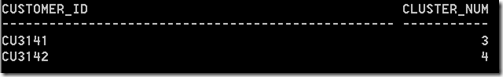Is we look back to Step 3 above we will see that the clusters listed correspond to what we have discovered.

The next function is CLUSTER_PROBABILTY. With this function we can see what the probability of customer belonging to a particular cluster. Using the results for customer CU3141 we can see what the probability is for this cluster, along with a few other clusters.

SELECT customer_id,
cluster_probability(cluster_kmeans_model, '3' USING *) as Cluster_3_Prob,
cluster_probability(cluster_kmeans_model, '4' USING *) as Cluster_4_Prob,
cluster_probability(cluster_kmeans_model, '7' USING *) as Cluster_7_Prob,
cluster_probability(cluster_kmeans_model, '9' USING *) as Cluster_9_Prob
FROM   insurance_cust_ltv
WHERE  customer_id = 'CU3141';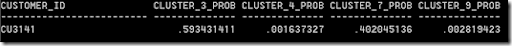We can also combine the CLUSTER_ID and CLUSER_PROBABILITY functions in one SELECT statement.

In the following query we want to know what the most likely cluster is for two customers and the cluster probability.

SELECT customer_id,
cluster_id(cluster_kmeans_model USING *) as Cluster_Num,
cluster_probability(cluster_kmeans_model, cluster_id(cluster_kmeans_model USING *) USING *) as Cluster_Prob
FROM   insurance_cust_ltv
WHERE  customer_id in ('CU3141', 'CU3142');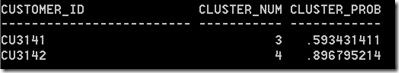Check back soon for my more blog posts on performing data mining in Oracle, using the Oracle Data Miner tool (part of SQL Developer) and the in-database SQL and PL/SQL code.

I hope you have enjoyed blog posts on Oracle Data Miner and you have found them useful. Let me know if there are specific topics you would like me to cover.

Thanks

Brendan Tierney